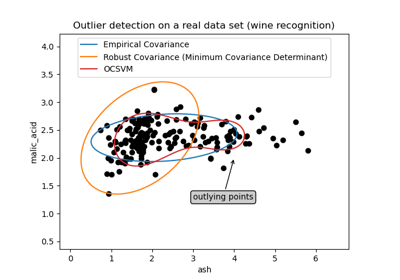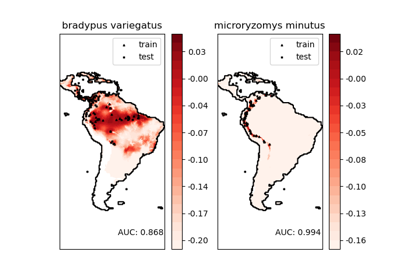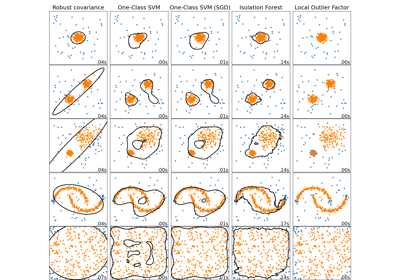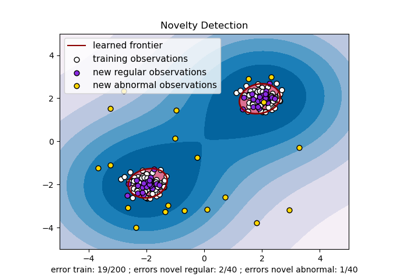# sklearn.svm.OneClassSVM¶

class sklearn.svm.OneClassSVM(*, kernel='rbf', degree=3, gamma='scale', coef0=0.0, tol=0.001, nu=0.5, shrinking=True, cache_size=200, verbose=False, max_iter=- 1)[source]

Unsupervised Outlier Detection.

Estimate the support of a high-dimensional distribution.

The implementation is based on libsvm.

Read more in the User Guide.

Parameters
kernel{‘linear’, ‘poly’, ‘rbf’, ‘sigmoid’, ‘precomputed’}, default=’rbf’

Specifies the kernel type to be used in the algorithm. It must be one of ‘linear’, ‘poly’, ‘rbf’, ‘sigmoid’, ‘precomputed’ or a callable. If none is given, ‘rbf’ will be used. If a callable is given it is used to precompute the kernel matrix.

degreeint, default=3

Degree of the polynomial kernel function (‘poly’). Ignored by all other kernels.

gamma{‘scale’, ‘auto’} or float, default=’scale’

Kernel coefficient for ‘rbf’, ‘poly’ and ‘sigmoid’.

• if gamma='scale' (default) is passed then it uses 1 / (n_features * X.var()) as value of gamma,

• if ‘auto’, uses 1 / n_features.

Changed in version 0.22: The default value of gamma changed from ‘auto’ to ‘scale’.

coef0float, default=0.0

Independent term in kernel function. It is only significant in ‘poly’ and ‘sigmoid’.

tolfloat, default=1e-3

Tolerance for stopping criterion.

nufloat, default=0.5

An upper bound on the fraction of training errors and a lower bound of the fraction of support vectors. Should be in the interval (0, 1]. By default 0.5 will be taken.

shrinkingbool, default=True

Whether to use the shrinking heuristic. See the User Guide.

cache_sizefloat, default=200

Specify the size of the kernel cache (in MB).

verbosebool, default=False

Enable verbose output. Note that this setting takes advantage of a per-process runtime setting in libsvm that, if enabled, may not work properly in a multithreaded context.

max_iterint, default=-1

Hard limit on iterations within solver, or -1 for no limit.

Attributes
class_weight_ndarray of shape (n_classes,)

Multipliers of parameter C for each class. Computed based on the class_weight parameter.

coef_ndarray of shape (1, n_features)

Weights assigned to the features (coefficients in the primal problem). This is only available in the case of a linear kernel.

coef_ is readonly property derived from dual_coef_ and support_vectors_.

dual_coef_ndarray of shape (1, n_SV)

Coefficients of the support vectors in the decision function.

fit_status_int

0 if correctly fitted, 1 otherwise (will raise warning)

intercept_ndarray of shape (1,)

Constant in the decision function.

n_support_ndarray of shape (n_classes,), dtype=int32

Number of support vectors for each class.

offset_float

Offset used to define the decision function from the raw scores. We have the relation: decision_function = score_samples - offset_. The offset is the opposite of intercept_ and is provided for consistency with other outlier detection algorithms.

New in version 0.20.

shape_fit_tuple of int of shape (n_dimensions_of_X,)

Array dimensions of training vector X.

support_ndarray of shape (n_SV,)

Indices of support vectors.

support_vectors_ndarray of shape (n_SV, n_features)

Support vectors.

Examples

>>> from sklearn.svm import OneClassSVM
>>> X = [, [0.44], [0.45], [0.46], ]
>>> clf = OneClassSVM(gamma='auto').fit(X)
>>> clf.predict(X)
array([-1,  1,  1,  1, -1])
>>> clf.score_samples(X)
array([1.7798..., 2.0547..., 2.0556..., 2.0561..., 1.7332...])


Methods

 Signed distance to the separating hyperplane. fit(X[, y, sample_weight]) Detects the soft boundary of the set of samples X. fit_predict(X[, y]) Perform fit on X and returns labels for X. get_params([deep]) Get parameters for this estimator. Perform classification on samples in X. Raw scoring function of the samples. set_params(**params) Set the parameters of this estimator.
decision_function(X)[source]

Signed distance to the separating hyperplane.

Signed distance is positive for an inlier and negative for an outlier.

Parameters
Xarray-like of shape (n_samples, n_features)

The data matrix.

Returns
decndarray of shape (n_samples,)

Returns the decision function of the samples.

fit(X, y=None, sample_weight=None, **params)[source]

Detects the soft boundary of the set of samples X.

Parameters
X{array-like, sparse matrix} of shape (n_samples, n_features)

Set of samples, where n_samples is the number of samples and n_features is the number of features.

sample_weightarray-like of shape (n_samples,), default=None

Per-sample weights. Rescale C per sample. Higher weights force the classifier to put more emphasis on these points.

yIgnored

not used, present for API consistency by convention.

Returns
selfobject

Notes

If X is not a C-ordered contiguous array it is copied.

fit_predict(X, y=None)[source]

Perform fit on X and returns labels for X.

Returns -1 for outliers and 1 for inliers.

Parameters
X{array-like, sparse matrix, dataframe} of shape (n_samples, n_features)
yIgnored

Not used, present for API consistency by convention.

Returns
yndarray of shape (n_samples,)

1 for inliers, -1 for outliers.

get_params(deep=True)[source]

Get parameters for this estimator.

Parameters
deepbool, default=True

If True, will return the parameters for this estimator and contained subobjects that are estimators.

Returns
paramsmapping of string to any

Parameter names mapped to their values.

predict(X)[source]

Perform classification on samples in X.

For a one-class model, +1 or -1 is returned.

Parameters
X{array-like, sparse matrix} of shape (n_samples, n_features) or (n_samples_test, n_samples_train)

For kernel=”precomputed”, the expected shape of X is (n_samples_test, n_samples_train).

Returns
y_predndarray of shape (n_samples,)

Class labels for samples in X.

score_samples(X)[source]

Raw scoring function of the samples.

Parameters
Xarray-like of shape (n_samples, n_features)

The data matrix.

Returns
score_samplesndarray of shape (n_samples,)

Returns the (unshifted) scoring function of the samples.

set_params(**params)[source]

Set the parameters of this estimator.

The method works on simple estimators as well as on nested objects (such as pipelines). The latter have parameters of the form <component>__<parameter> so that it’s possible to update each component of a nested object.

Parameters
**paramsdict

Estimator parameters.

Returns
selfobject

Estimator instance.

## Examples using sklearn.svm.OneClassSVM¶